Fundamental Parameters Of Traffic Flow - 1

# Fundamental Parameters Of Traffic Flow - 1 - Notes | Study Transportation Engineering - Civil Engineering (CE)

 1 Crore+ students have signed up on EduRev. Have you?

TRAFFIC STREAM PARAMETERS

• The traffic stream includes a combination of driver and vehicle behavior. The driver or human behavior being non-uniform, traffic stream is also non-uniform in nature. It is influenced not only by the individual characteristics of both vehicle and human but also by the way a group of such units interacts with each other. Thus a flow of traffic through a street of defined characteristics will vary both by location and time corresponding to the changes in the human behavior.
• The traffic engineer, but for the purpose of planning and design, assumes that these changes are within certain ranges which can be predicted. For example, if the maximum permissible speed of a highway is 60 kmph, the whole traffic stream can be assumed to move on an average speed of 40 kmph rather than 100 or 20 kmph. Thus the traffic stream itself is having some parameters on which the characteristics can be predicted.
The parameters can be mainly classified as: measurements of quantity, which includes density and flow of traffic and measurements of quality which includes speed. The traffic stream parameters can be macroscopic which characterizes the traffic as a whole or microscopic which studies the behavior of individual vehicle in the stream with respect to each other.
• As far as the macroscopic characteristics are concerned, they can be grouped as measurement of quantity or quality as described above, i.e. flow, density, and speed. While the microscopic characteristics include the measures of separation, i.e. the headway or separation between vehicles which can be either time or space headway. The fundamental stream characteristics are speed, flow, and density and are discussed below.

SPEED
Speed is considered as a quality measurement of travel as the drivers and passengers will be concerned more about the speed of the journey than the design aspects of the traffic. It is defined as the rate of motion in distance per unit of time. Mathematically speed or velocity v is given by,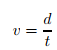(1.1)
where, v is the speed of the vehicle in m/s, d is distance traveled in m in time t seconds. Speed of different vehicles will vary with respect to time and space. To represent these variation, several types of speed can be defined. Important among them are spot speed, running speed, journey speed, time mean speed and space mean speed. These are discussed below:

Spot Speed
Spot speed is the instantaneous speed of a vehicle at a specified location. Spot speed can be used to design the geometry of road like horizontal and vertical curves, super elevation etc. Location and size of signs, design of signals, safe speed, and speed zone determination, require the spot speed data. Accident analysis, road maintenance, and congestion are the modern fields of traffic engineer, which uses spot speed data as the basic input. Spot speed can be measured using an enoscope, pressure contact tubes or direct timing procedure or radar speedometer or by time-lapse photographic methods. It can be determined by speeds extracted from video images by recording the distance travelling by all vehicles between a particular pair of frames.

Running Speed
Running speed is the average speed maintained over a particular course while the vehicle is moving and is found by dividing the length of the course by the time duration the vehicle was in motion. i.e. this speed doesn’t consider the time during which the vehicle is brought to a stop, or has to wait till it has a clear road ahead. The running speed will always be more than or equal to the journey speed, as delays are not considered in calculating the running speed

Journey Speed
Journey speed is the effective speed of the vehicle on a journey between two points and is the distance between the two points divided by the total time taken for the vehicle to complete the journey including any stopped time. If the journey speed is less than running speed, it indicates that the journey follows a stop-go condition with enforced acceleration and deceleration. The spot speed here may vary from zero to some maximum in excess of the running speed. A uniformity between journey and running speeds denotes comfortable travel conditions.

TIME MEAN SPEED AND SPACE MEAN SPEED
Time mean speed is defined as the average speed of all the vehicles passing a point on a highway over some specified time period. Space mean speed is defined as the average speed of all the vehicles occupying a given section of a highway over some specified time period. Both mean speeds will always be different from each other except in the unlikely event that all vehicles are traveling at the same speed. Time mean speed is a point measurement while space mean speed is a measure relating to length of highway or lane, i.e. the mean speed of vehicles over a period of time at a point in space is time mean speed and the mean speed over a space at a given instant is the space mean speed.

FLOW/VOLUME
There are practically two ways of counting the number of vehicles on a road. One is flow or volume, which is defined as the number of vehicles that pass a point on a highway or a given lane or direction of a highway during a specific time interval. The measurement is carried out by counting the number of vehicles, nt , passing a particular point in one lane in a defined period t. Then the flow q expressed in vehicles/hour is given by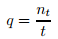(1.2)
Flow is expressed in planning and design field taking a day as the measurement of time.

Variations Of Volume
The variation of volume with time, i.e. month to month, day to day, hour to hour and within a hour is also as important as volume calculation. Volume variations can also be observed from season to season. Volume will be above average in a pleasant motoring month of summer, but will be more pronounced in rural than in urban area. But this is the most consistent of all the variations and affects the traffic stream characteristics the least.
Weekdays, Saturdays and Sundays will also face difference in pattern. But comparing day with day, patterns for routes of a similar nature often show a marked similarity, which is useful in enabling predictions to be made.
The most significant variation is from hour to hour. The peak hour observed during mornings and evenings of weekdays, which is usually 8 to 10 per cent of total daily flow or 2 to 3 times the average hourly volume. These trips are mainly the work trips, which are relatively stable with time and more or less constant from day to day.

Types Of Volume Measurements
Since there is considerable variation in the volume of traffic, several types of measurements of volume are commonly adopted which will average these variations into a single volume count to be used in many design purposes.
1. Average Annual Daily Traffic(AADT): The average 24-hour traffic volume at a given location over a full 365-day year, i.e. the total number of vehicles passing the site in a year divided by 365.
2. Average Annual Weekday Traffic(AAWT): The average 24-hour traffic volume occurring on weekdays over a full year. It is computed by dividing the total weekday traffic volume for the year by 260.
3. Average Daily Traffic(ADT): An average 24-hour traffic volume at a given location for some period of time less than a year. It may be measured for six months, a season, a month, a week, or as little as two days. An ADT is a valid number only for the period over which it was measured.
4. Average Weekday Traffic(AWT): An average 24-hour traffic volume occurring on weekdays for some period of time less than one year, such as for a month or a season.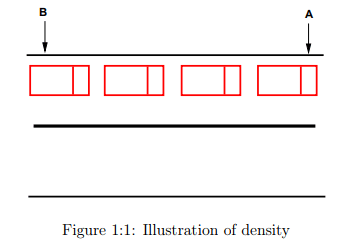The relationship between AAWT and AWT is analogous to that between AADT and ADT. Volume in general is measured using different ways like manual counting, detector/sensor counting, moving-car observer method, etc. Mainly the volume study establishes the importance of a particular route with respect to the other routes, the distribution of traffic on road, and the fluctuations in flow. All which eventually determines the design of a highway and the related facilities. Thus, volume is treated as the most important of all the parameters of traffic stream.

DENSITY
Density is defined as the number of vehicles occupying a given length of highway or lane and is generally expressed as vehicles per km. One can photograph a length of road x, count the number of vehicles, nx, in one lane of the road at that point of time and derive the density k as,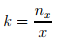(1.3)
This is illustrated in figure 1:1. From the figure, the density is the number of vehicles between the point A and B divided by the distance between A and B. Density is also equally important as flow but from a different angle as it is the measure most directly related to traffic demand. Again it measures the proximity of vehicles in the stream which in turn affects the freedom to maneuver and comfortable driving.

DERIVED CHARACTERISTICS
From the fundamental traffic flow characteristics like flow, density, and speed, a few other parameters of traffic flow can be derived. Significant among them are the time headway,distance headway and travel time. They are discussed one by one below:

The microscopic character related to volume is the time headway or simply headway. Time headway is defined as the time difference between any two successive vehicles when they cross a given point. Practically, it involves the measurement of time between the passage of one rear bumper and the next past a given point. If all headways h in time period, t, over which flow has been measured are added then,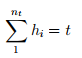(1.4)
But the flow is defined as the number of vehicles nt measured in time interval t, that is,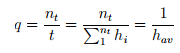(1.5)
where, hav is the average headway. Thus average headway is the inverse of flow. Time headway is often referred to as simply the headway.

Another related parameter is the distance headway. It is defined as the distance between corresponding points of two successive vehicles at any given time. It involves the measurement from a photograph, the distance from rear bumper of lead vehicle to rear bumper of following vehicle at a point of time. If all the space headways in distance x over which the density has been measured are added,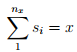(1.6)
But the density (k) is the number of vehicles nx at a distance of x, that is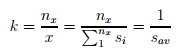(1.7)
Where, sav is average distance headway. The average distance headway is the inverse of density and is sometimes called as spacing

Travel Time
Travel time is defined as the time taken to complete a journey. As the speed increases, travel time required to reach the destination also decreases and vice-versa. Thus travel time is inversely proportional to the speed. However, in practice, the speed of a vehicle fluctuates over time and the travel time represents an average measure.

TIME-SPACE DIAGRAM
Time space diagram is a convenient tool in understanding the movement of vehicles. It shows the trajectory of vehicles in the form of a two dimensional plot. Time space diagram can be plotted for a single vehicle as well as multiple vehicles. They are discussed below:

Single Vehicle
Taking one vehicle at a time, analysis can be carried out on the position of the vehicle with respect to time. This analysis will generate a graph which gives the relation of its position on a road stretch relative to time. This plot thus will be between distance x and time t and x will be a functions the position of the vehicle for every t along the road stretch. This graphical representation of x(t) in a (t, x) plane is a curve which is called as a trajectory. The trajectory provide an intuitive, clear, and complete summary of vehicular motion in one dimension.
In figure 1:2(a), the the distance x goes on increasing with respect to the origin as time progresses. The vehicle is moving at a smooth condition along the road way. In figure 1:2(b), the vehicle at first moves with a smooth pace after reaching a position reverses its direction of movement. In figure 1:2(c), the vehicle in between becomes stationary and maintains the same position.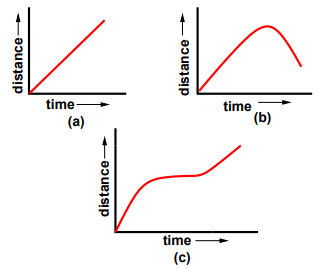Figure 1:2: Time space diagram for a single vehicle

From the figure, steeply increasing section of x(t) denote a rapidly advancing vehicle and horizontal portions of x(t) denote a stopped vehicle while shallow sections show a slow-moving vehicle. A straight line denotes constant speed motion and curving sections denote accelerated motion; and if the curve is concave downwards it denotes acceleration. But a curve which is convex upwards denotes deceleration

Multiple Vehicles
Time-space diagram can also be used to determine the fundamental parameters of traffic flow like speed, density and volume. It can also be used to find the derived characteristics like space headway and time headway. Figure 1:3 shows the time-space diagram for a set of vehicles traveling at constant speed. Density, by definition is the number of vehicles per unit length. From the figure, an observer looking into the stream can count 4 vehicles passing the stretch of road between x1 and x2 at time t. Hence, the density is given as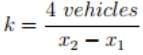(1.8)

We can also find volume from this time-space diagram. As per the definition, volume is the number of vehicles counted for a particular interval of time. From the figure 1:3 we can see that 6 vehicles are present between the time t1 and t2. Therefore, the volume q is given as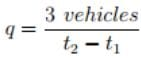(1.9)
Again the averages taken at a specific location (i.e., time ranging over an interval) are called time means and those taken at an instant over a space interval are termed as space means. Another related definition which can be given based on the time-space diagram is the headway. Space headway is defined as the distance between corresponding points of two successive vehicles at any given time. Thus, the vertical gap between any two consecutive lines represents space headway. The reciprocal of density otherwise gives the space headway between vehicles at that time.
Similarly, time headway is defined as the time difference between any two successive vehicles when they cross a given point. Thus, the horizontal gap between the vehicles represented by the lines gives the time headway. The reciprocal of flow gives the average time headway between vehicles at that point.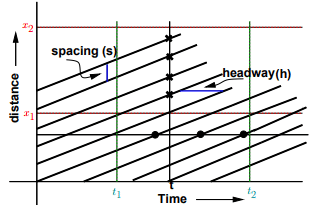Figure 1:3: Time space diagram for many vehicles

The document Fundamental Parameters Of Traffic Flow - 1 - Notes | Study Transportation Engineering - Civil Engineering (CE) is a part of the Civil Engineering (CE) Course Transportation Engineering.
All you need of Civil Engineering (CE) at this link: Civil Engineering (CE)

## Transportation Engineering

22 videos|68 docs|58 tests

## Transportation Engineering

22 videos|68 docs|58 tests

Track your progress, build streaks, highlight & save important lessons and more!

,

,

,

,

,

,

,

,

,

,

,

,

,

,

,

,

,

,

,

,

,

;